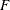TEST Statement

TEST <H=effects> E=effect </ options> ;

By default, for each sum of squares in the analysis an F value is computed that uses the residual MS as an error term. Use a TEST statement to request additional F tests that use other effects as error terms. You need a TEST statement when a nonstandard error structure (as in a split-plot design) exists. Note, however, that this might not be appropriate if the design is unbalanced, since in most unbalanced designs with nonstandard error structures, mean squares are not necessarily independent with equal expectations under the null hypothesis.

Caution: The GLM procedure does not check any of the assumptions underlying the F statistic. When you specify a TEST statement, you assume sole responsibility for the validity of thestatistic produced. To help validate a test, you can use the RANDOM statement and inspect the expected mean squares, or you can use the TEST option of the RANDOM statement.

You can use as many TEST statements as you want, provided that they appear after the MODEL statement.

You can specify the following terms in the TEST statement.

H=effects

specifies which effects in the preceding model are to be used as hypothesis (numerator) effects.

E=effect

specifies one, and only one, effect to use as the error (denominator) term. The E= specification is required.

By default, the sum of squares type for all hypothesis sum of squares and error sum of squares is the highest type computed in the model. If the hypothesis type or error type is to be another type that was computed in the model, you should specify one or both of the following options after a slash (/).

ETYPE=n

specifies the type of sum of squares to use for the error term. The type must be a type computed in the model (n=1, 2, 3, or 4 ).

HTYPE=n

specifies the type of sum of squares to use for the hypothesis. The type must be a type computed in the model (n=1, 2, 3, or 4).

This example illustrates the TEST statement with a split-plot model:

proc glm;
class a b c;
model y=a  b(a) c a*c b*c(a);
test h=a e=b(a)/ htype=1 etype=1;
test h=c a*c e=b*c(a) / htype=1 etype=1;
run;